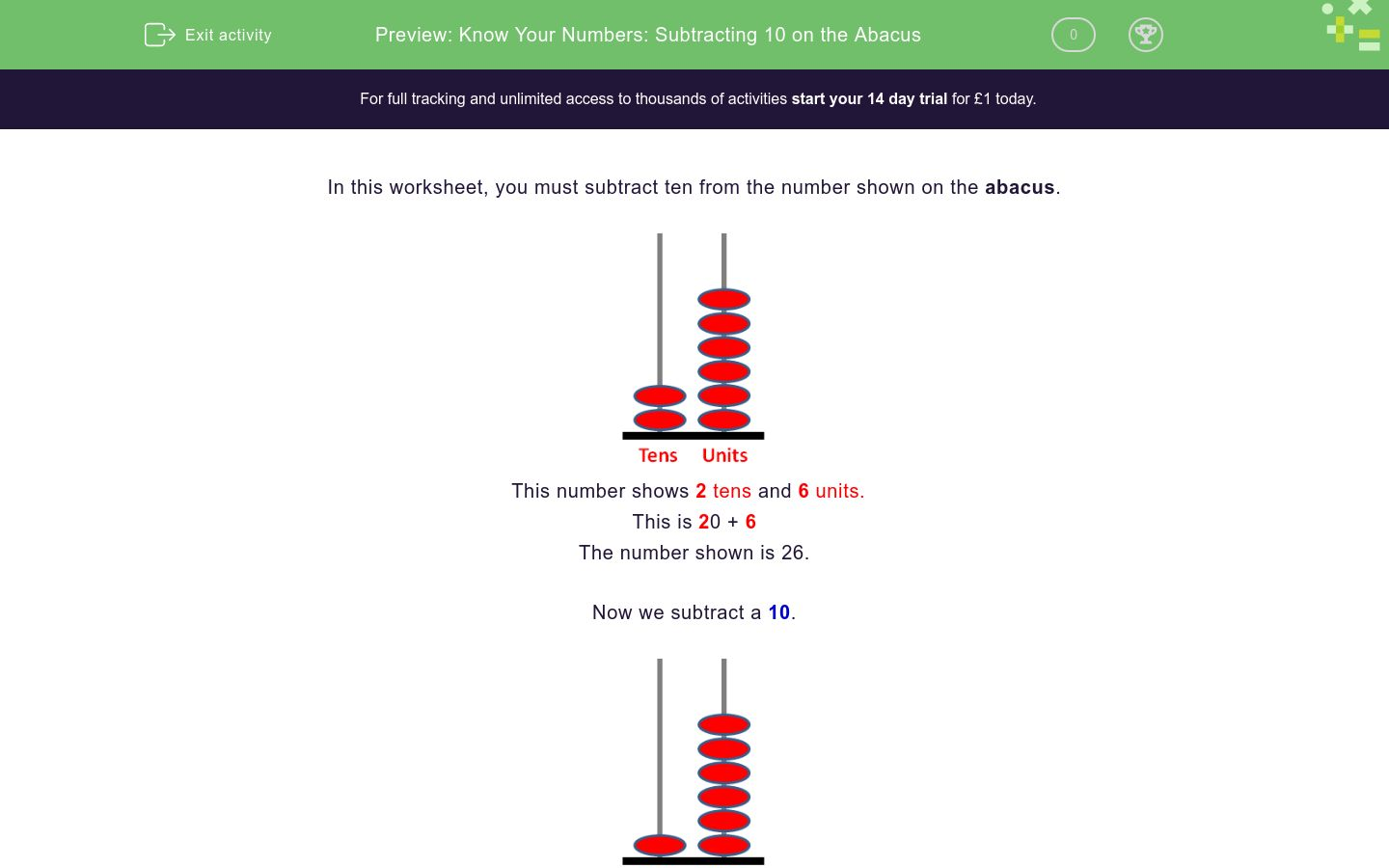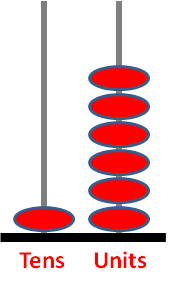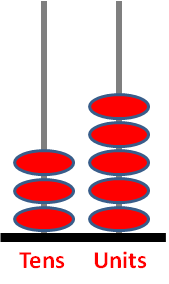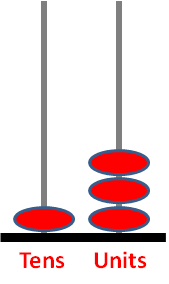# Know Your Numbers: Subtracting 10 on the Abacus

In this worksheet, students subtract 10 from the number shown on the tens and units abacus.Key stage:  KS 1

Curriculum topic:   Number: Addition and Subtraction

Curriculum subtopic:   Add and Subtract to 20

Difficulty level:### QUESTION 1 of 10

In this worksheet, you must subtract ten from the number shown on the abacus.This number shows 2 tens and 6 units.

This is 20 + 6

The number shown is 26.

Now we subtract a 10.One less ten makes 1 ten and 6 units so the answer is 16.

Subtract 10 from the number shown on this abacus.Subtract 10 from the number shown on this abacus.Subtract 10 from the number shown on this abacus.Subtract 10 from the number shown on this abacus.Subtract 10 from the number shown on this abacus.Subtract 10 from the number shown on this abacus.Subtract 10 from the number shown on this abacus.Subtract 10 from the number shown on this abacus.Subtract 10 from the number shown on this abacus.Subtract 10 from the number shown on this abacus.• Question 1

Subtract 10 from the number shown on this abacus.33
EDDIE SAYS
4 tens − 1 ten = 3 tens
3 tens + 3 units = 33
• Question 2

Subtract 10 from the number shown on this abacus.65
EDDIE SAYS
7 tens − 1 ten = 6 tens
6 tens + 5 units = 65
• Question 3

Subtract 10 from the number shown on this abacus.11
EDDIE SAYS
2 tens − 1 ten = 1 ten
1 ten + 1 unit = 11
• Question 4

Subtract 10 from the number shown on this abacus.10
EDDIE SAYS
2 tens − 1 ten = 10
There are no units.
• Question 5

Subtract 10 from the number shown on this abacus.16
EDDIE SAYS
2 tens − 1 ten = 1 ten
1 ten + 6 units = 16
• Question 6

Subtract 10 from the number shown on this abacus.25
EDDIE SAYS
3 tens − 1 ten = 2 tens
2 tens + 5 units = 25
• Question 7

Subtract 10 from the number shown on this abacus.56
EDDIE SAYS
6 tens − 1 ten = 5 tens
5 tens + 6 units = 56
• Question 8

Subtract 10 from the number shown on this abacus.73
EDDIE SAYS
8 tens − 1 ten = 7 tens
7 tens + 3 units = 73
• Question 9

Subtract 10 from the number shown on this abacus.28
EDDIE SAYS
3 tens − 1 ten = 2 tens
2 tens + 8 units + 28
• Question 10

Subtract 10 from the number shown on this abacus.3
EDDIE SAYS
1 ten − 1 ten = no tens
no tens + 3 units = 3
---- OR ----

Sign up for a £1 trial so you can track and measure your child's progress on this activity.

### What is EdPlace?

We're your National Curriculum aligned online education content provider helping each child succeed in English, maths and science from year 1 to GCSE. With an EdPlace account you’ll be able to track and measure progress, helping each child achieve their best. We build confidence and attainment by personalising each child’s learning at a level that suits them.

Get started